MORE IN Structural Analysis - 1
GTU Civil Engineering (Semester 3)
Structural Analysis - 1
May 2015
Total marks: --
Total time: --
INSTRUCTIONS
(1) Assume appropriate data and state your reasons
(2) Marks are given to the right of every question
(3) Draw neat diagrams wherever necessary

1 (a) State basic difference between fixed and simply supported beams. State advantages of fixed beam over simply supported beam.
7 M
1 (b) Find the torque which a shaft of 50 mm diameter can transmit safely, if the shear stress is not to exceed 50 N/mm2 .
7 M

2 (a) Find slope & deflection for the following structure as shown in fig.1 by double integration method. Take E=200 kN/mm2 and I=14400 × 104mm4.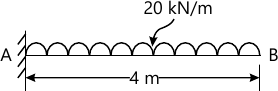7 M
2 (b) What is an influence line diagram? Explain its importance in structural analysis?
7 M
2 (c) Give equations of Static and Kinematics Indeterminacy for the following structures with meaning of each term used.
(i) Beam
(ii) Grid
(iii) Plane Frame
(iv) plane truss
7 M

3 (a) A fixed beam AB of span L carried a UDL of w per meter length over entire span. Support B settles during application of load. Calculate the settlement, so that there is no fixed end moment at B. Also find FEM at A.
7 M
3 (b) A strut 2.5m long is 60mm in diameter. One end of the strut is fixed while its other end is hinged. Find the compressive load for the member using Euler's formula, allowing a factor of safety of 3.5. Take E=2.1 × 105 N/mm2 .
7 M
3 (c) A three hinged parabolic arch has a span of 30m and a rise to the central hinge of 5m. The arch is loaded with two vertical loads of 200kN symmetrically situated on either side of the central hinge at 3.75m horizontally from the hinge. Calculate the maximum positive and negative bending moments in the arch stating where they occur.
7 M
3 (d) Draw influence line diagram for the parameters shown in fig.2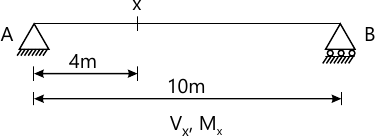7 M

4 (a) Find slope at point 'A' and 'B' using Macaulay's method. Refer fig:3. Take E=210 GPa and I=16000 × 104 mm4.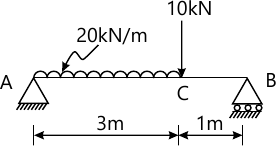10 M
4 (b) Differentiate between real beam and conjugate beam. Justify the support condition in conjugate beam.
4 M
4 (c) A masonry wall 6 meter high, is of solid rectangular section, 4 meter wide and 2 meter thick. A horizontal wind pressure of 1.3 kN/m2 acts on 4 meter side. Find the maximum and minimum stresses induced on the base, if the unit weight of masonry is 22.4 kN/m3
10 M
4 (d) Calculate value of maximum limit of eccentricity for circular section.
4 M

5 (a) Find the diameter of shaft required to transmit 160KW at 250 RPM, if the maximum torque is not to exceed the mean torque by 30% with a maximum permissible shear stress of 100 N/mm2.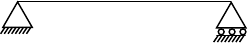7 M
5 (b) Find static and kinematic indeterminacy for the structures shown below. Also comment about stability.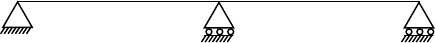7 M
5 (c) A hollow cast iron column 5m long is fixed at both ends and has an external diameter of 300mm. The column supports an axial load of 1200kN. Find the internal diameter of the column, adopting a factor of safety of 5. Take fc =550N/mm2 and α=1/1600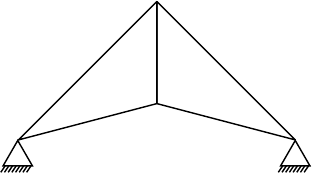7 M
5 (d) It is found that a bar 36mm in diameter stretches 1.9mm under a gradually applied load of 150kN. If a weight of 1500N is dropped on to a collar at the lower end of this bar, through a height of 50mm before commencing to stretch the bar, calculate the maximum instantaneous stress and elongation produced in the bar. E=215kN/mm2.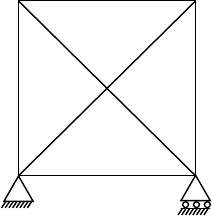7 M

More question papers from Structural Analysis - 1